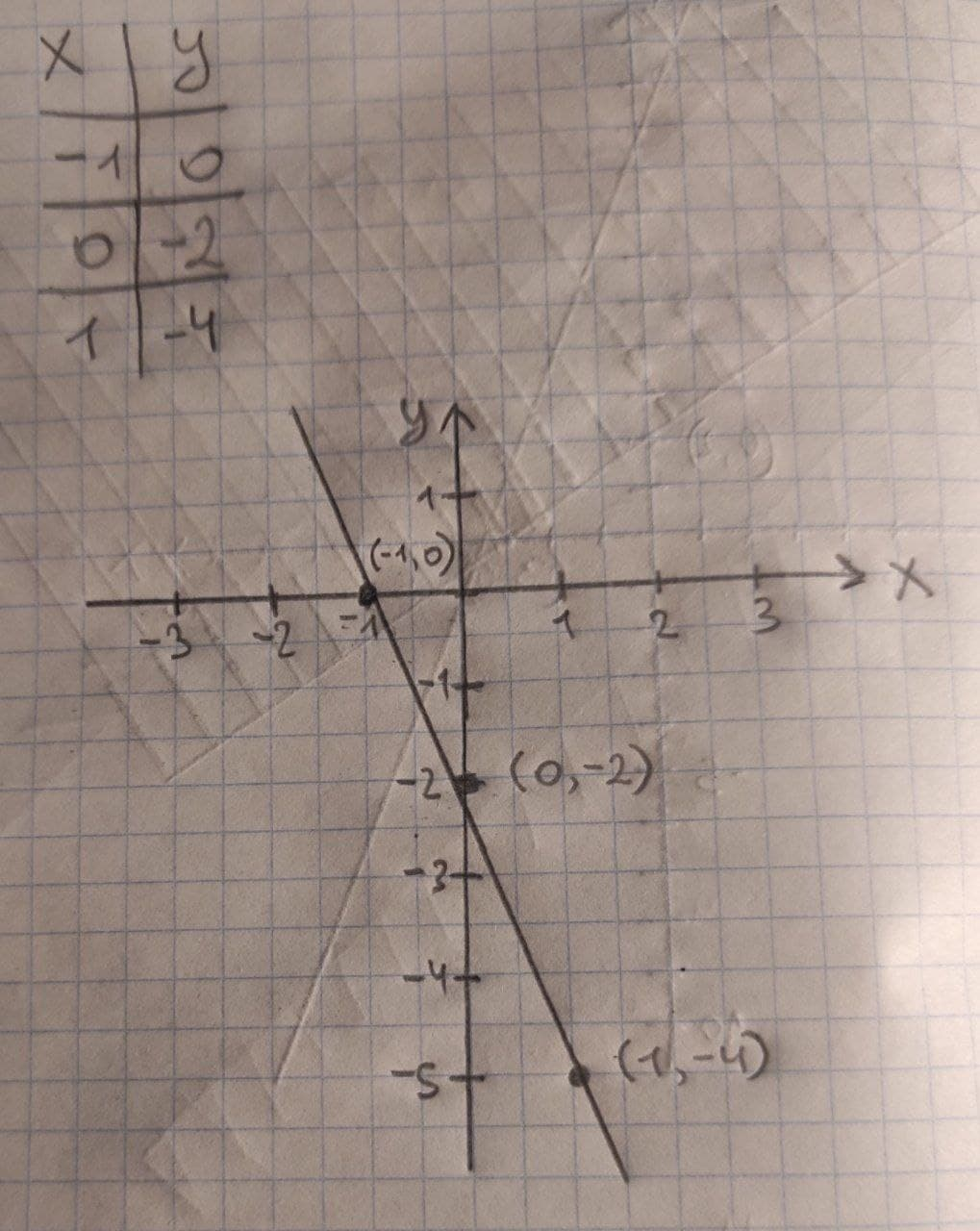# What would be the linear equation for this function, & the graph? (X_1,Y_1)=(-3,4), m=-2preprekomW 2021-03-18 Answered
What would be the linear equation for this function, & the graph?
$\left({X}_{1},{Y}_{1}\right)=\left(-3,4\right),m=-2$
You can still ask an expert for help

• Questions are typically answered in as fast as 30 minutes

Solve your problem for the price of one coffee

• Math expert for every subject
• Pay only if we can solve itunessodopunsep

To find the linear equation we need point slope formula.
Given slope $=-2$ and point $=\left({x}_{1},{y}_{1}\right)=\left(-3,4\right)$
$y-{y}_{1}=m\left(x-{x}_{!}\right)$
$y-4=-2\left(x-\left(-3\right)\right)$
$y-4=-2\left(x+3\right)$
$y-4=-2x-6$
$y=-2x-6+4$
$y=2x-2$
For $y=-2x-2$ we make a table.
Picked few random values for x, then found y for those x.Then plot the points and join using a line.